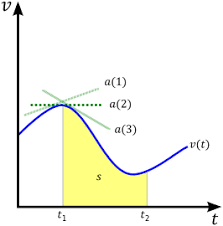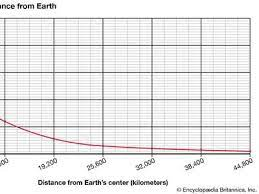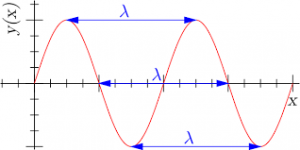## How to Calculate and Solve for Velocity | De Broglie’s LawThe image above represents velocity.

To compute for velocity, three essential parameters are needed and these parameters are Planck’s Constant (h), Wavelength (λ) and Mass (m).

The formula for calculating velocity:

v = h / λm

Where;

v = velocity
λ = wavelength
h = Planck’s constant
m = mass

Let’s solve an example;
Find the velocity when the wavelength is 10, the planck’s constant is 6.626e-34 and the mass is 5.

This implies that;

λ = wavelength = 10
h = Planck’s constant = 6.626e-34
m = mass = 5

v = h / λm
v = 6.62607004e-34 (10)(5)
v = 6.62607004e-34 (50)
v = 1.33e-35

Therefore, the velocity is 1.33e-35 m/s.

## How to Calculate and Solve for Mass | De Broglie’s LawThe image above represents mass.

To compute for mass, three essential parameters are needed and these parameters are Planck’s Constant (h), Wavelength (λ) and Velocity (v).

The formula for calculating the mass:

m = h / λv

Where;

m = mass
λ = wavelength
h = Planck’s constant
v = velocity

Let’s solve an example;
Find the mass when the wavelength is 4, the planck’s constant is 6.626e-34 and the velocity is 2.

This implies that;

λ = wavelength = 4
h = Planck’s constant = 6.626e-34
v = velocity = 2

m = hλv
m = 6.62607004e-34(4)(2)
m = 6.62607004e-34(8)
m = 8.28e-35

Therefore, the mass is 8.28e-35 kg.

## How to Calculate and Solve for Wavelength | De Broglie’s LawThe image above represents wavelength.

To compute for wavelength, three essential parameters are needed and these parameters are Planck’s Constant (h), Mass (m) and Velocity (v).

The formula for calculating wavelength:

λ = hmv

Where

λ = wavelength
h = Planck’s constant
m = mass
v = velocity

Let’s solve an example;
Find the wavelength when the planck’s constant is 6.62e-34, the mass is 21 and the velocity is 11.

This implies that;

h = Planck’s constant = 6.62e-34
m = mass = 21
v = velocity = 11

λ = hmv
λ = 6.62607004e-34(21)(11)
λ = 6.62607004e-34(231)
λ = 2.86e-36

Therefore, the wavelength is 2.86e-36 m.

Calculating the Mass when the Wavelength, the Planck’s Constant and the Velocity is Given.

m = h / λv

Where;

m = mass
λ = wavelength
h = Planck’s constant
v = velocity

Let’s solve an example;
Find the mass when the wavelength is 18, the planck’s constant is 6.626e-34 and the velocity is 6.

This implies that;

λ = wavelength = 18
h = Planck’s constant = 6.626e-34
v = velocity = 6

m = h / λv
m = 18 / 6.626e-34 x 6
m = 18 / 3.976e-33
m = 4.53

Therefore, the mass is 4.53 m.